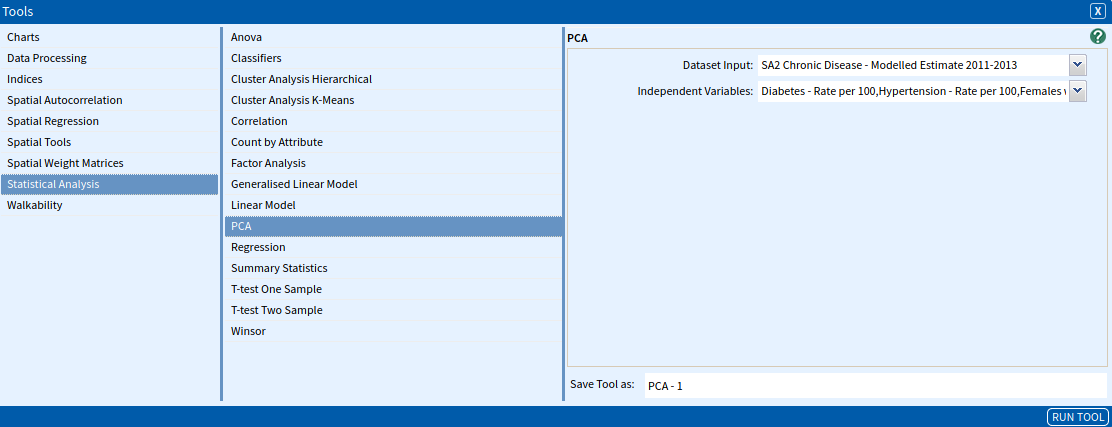# Principal Component Analysis (PCA)

Principal Component Analysis (PCA) is a multivariate analysis technique to transform a set of variables into a smaller set of uncorrelated components that accounts for the maximum variance in the data set. The calculation in R is done by a singular value decomposition (SVD) of the (centred and possibly scaled) data matrix, not by using Eigen on the covariance matrix. This is generally the preferred method for numerical accuracy.

Because of the complexity of PCA formula, no formula is provided in this section. Please refer to the references at the end of this guide for further information.

### SET UP

For this worked example, we will generate analysis of common diseases in Melbourne.

• Select Greater Melbourne as your area.
• Select SA2 Chronic Disease – Modelled Estimate 2011-2013 as your dataset, selecting the following variables:
• Statistical Area Level 2 Code
• Diabetes – Rate per 100
• Hypertension – Rate per 100
• Females with Mental and Behavioural Problems – Rate per 100

### Inputs

Once you have set up the area and dataset, open the PCA tool (Tools → Statistical Analysis → PCA) and enter the parameters as described below.

• Dataset Input: The dataset that contains the variables of interest. Select SA2 Chronic Disease – Modelled Estimate 2011-2013.
• Independent Variables: The set of independent variables that you would like to analyse. Select the following variables:
• Diabetes – Rate per 100
• Hypertension – Rate per 100
• Females with Mental and Behavioural Problems – Rate per 100Once you have entered your parameters, click Run Tool.

### Outputs

Once you have run the tool, click on the Display Output button in the pop-up window that appears. This will bring up a simple text window like the one below.The outputs of the PCA tool provides the following:

• Rotation Matrix: The matrix of variable loadings (i.e., a matrix whose columns contain the eigenvectors).
• Center: The means that were subtracted.
• Scale: The scalings that have been applied to each variable.
• Predict PCA X: A numeric matrix or data frame which provides the data for the principal components analysis. This has been cut in size for the above example.
• Standard deviation: The standard deviations of the principal components.
• Proportion of Variance: Percent variance explained individually.
• Cumulative Proportion: Percent variance explained cumulatively.
Dunteman, G. H. (1989). Principal components analysis. Sage.
Jolliffe, I. T. (2002). Principal components in regression analysis. Principal Component Analysis, 167–198.
Sánchez, J., Mardia, K. V., Kent, J. T., & Bibby, J. M. (1979). Multivariate analysis. Academic Press, London-New York-Toronto-Sydney-San Francisco.

### Looking for Spatial Data?

You can browse the AURIN Data Discovery:### How can you Create Impact?